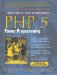# Section A.18. Math

### A.18. Math

#### A.18.1. Math_Basex

Simple class for converting base set of numbers with a customizable character base set.

##### A.18.1.1 Description

Base X conversion class

#### A.18.2. Math_Complex

Classes that define complex numbers and their operations

##### A.18.2.1 Description

Classes that represent and manipulate complex numbers. Contain definitions for basic arithmetic functions, as well as trigonometric, inverse trigonometric, hyperbolic, inverse hyperbolic, exponential and logarithms of complex numbers.

#### A.18.3. Math_Fibonacci

Package to calculat and manipulate Fibonacci numbers

##### A.18.3.1 Description

The Fibonacci series is constructed using the formula: F(n) = F(n - 1) + F (n - 2), By convention F(0) = 0, and F(1) = 1. An alternative formula that uses the Golden Ratio can also be used: F(n) = (PHI^n - phi^n)/sqrt(5) [Lucas' formula], where PHI = (1 + sqrt(5))/2 is the Golden Ratio, and phi = (1 - sqrt(5))/2 is its reciprocal Requires Math_Integer, and can be used with big integers if the GMP or the BCMATH libraries are present.

#### A.18.4. Math_Histogram

Classes to calculate histogram distributions

##### A.18.4.1 Description

Classes to calculate histogram distributions and associated statistics. Supports simple and cummulative histograms. You can generate regular (2D) histograms, 3D, or 4D histograms Data must not have nulls. Requires Math_Stats.

#### A.18.5. Math_Integer

Package to represent and manipulate integers

##### A.18.5.1 Description

The class Math_Integer can represent integers bigger than the signed longs that are the default of PHP, if either the GMP or the BCMATH (bundled with PHP) are present. Otherwise it will fall back to the internal integer representation. The Math_IntegerOp class defines operations on Math_Integer objects.

#### A.18.6. Math_Matrix

Class to represent matrices and matrix operations

##### A.18.6.1 Description

Matrices are represented as 2 dimensional arrays of numbers. This class defines methods for matrix objects, as well as static methods to read, write and manipulate matrices, including methods to solve systems of linear equations (with and without iterative error correction). Requires the Math_Vector package. For running the unit tests you will need PHPUnit version 0.6.2 or older.

#### A.18.7. Math_Numerical_RootFinding

Numerical analysis root finding methods package

##### A.18.7.1 Description

This package provide various numerical analysis methods for find root

Available Methods:

• Bisection

• False Position

• Fixed Point

• Newton-Raphson

• Secant

#### A.18.8. Math_Quaternion

Classes that define Quaternions and their operations

##### A.18.8.1 Description

Classes that represent and manipulate quaternions. Contain definitions for basic arithmetic functions in a static class. Quaternions are an extension of the idea of complex numbers, and a quaternion is defined as:

q = a + b*i + c*j + d*k

In 1844 Hamilton described a system in which numbers were composed of a real part and 3 imaginary and independent parts (i,j,k), such that:

i^2 = j^2 = k^2 = -1 and

ij = k, jk = i, ki = j and

ji = -k, kj = -i, ik = -j

The above are known as "Hamilton's rules"

#### A.18.9. Math_RPN

Reverse Polish Notation.

##### A.18.9.1 Description

Change Expression To RPN (Reverse Polish Notation) and evaluate it.

#### A.18.10. Math_Stats

Classes to calculate statistical parameters

##### A.18.10.1 Description

Package to calculate statistical parameters of numerical arrays of data. The data can be in a simple numerical array, or in a cummulative numerical array. A cummulative array, has the value as the index and the number of repeats as the value for the array item, e.g. \$data = array(3=>4, 2.3=>5, 1.25=>6, 0.5=>3). Nulls can be rejected, ignored or handled as zero values.

#### A.18.11. Math_TrigOp

Supplementary trigonometric functions

##### A.18.11.1 Description

Static class with methods that implement supplementary trigonometric, inverse trigonometric, hyperbolic, and inverse hyperbolic functions.

#### A.18.12. Math_Vector

Vector and vector operation classes

##### A.18.12.1 Description

Classes to represent Tuples, general Vectors, and 2D-/3D-vectors, as well as a static class for vector operations.PHP 5 Power Programming
ISBN: 013147149X
EAN: 2147483647
Year: 2003
Pages: 240

Similar book on Amazon